NCERT Solutions for Class 10 Maths Chapter 5- Arithmetic Progression Exercise 5.2 will give you a comprehensive methodology to solve nth term question of arithmetic progression. NCERT solutions will assist you in attaining perfection in all the methodologies provided in the textbook.

NCERT exercise solutions are presented here to ease your Class 10 second term exam preparation. This page covers all the answers to the questions provided in Exercise 5.2 of the NCERT Class 10 Maths textbook.

## Topics covered in Exercise 5.2

1. nth term of arithmetic progression
2. Solved examples
3. Alternate solutions

• Make you practise different types of questions.
• Helps you to know different methodologies to solve arithmetic progression.
• Boosts your confidence in solving arithmetic progression problems.
• It is the best study material to start your exam preparation.

### Access Answers of Maths NCERT Class 10 Chapter 5 – Arithmetic Progression Exercise 5.2

1. Fill in the blanks in the following table, given that a is the first term, d the common difference and an the nth term of the A.P.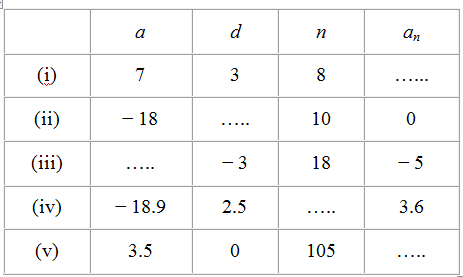Solutions:

(i) Given,

First term, a = 7

Common difference, d = 3

Number of terms, n = 8,

We have to find the nth term, an = ?

As we know, for an A.P.,

an = a+(n−1)d

Putting the values,

=> 7+(8 −1) 3

=> 7+(7) 3

=> 7+21 = 28

Hence, an = 28

(ii) Given,

First term, a = -18

Common difference, d = ?

Number of terms, n = 10

Nth term, an = 0

As we know, for an A.P.,

an = a+(n−1)d

Putting the values,

0 = − 18 +(10−1)d

18 = 9d

d = 18/9 = 2

Hence, common difference, = 2

(iii) Given,

First term, a = ?

Common difference, d = -3

Number of terms, n = 18

Nth term, an = -5

As we know, for an A.P.,

an = a+(n−1)d

Putting the values,

−5 = a+(18−1) (−3)

−5 = a+(17) (−3)

−5 = a−51

a = 51−5 = 46

Hence, a = 46

(iv) Given,

First term, a = -18.9

Common difference, d = 2.5

Number of terms, n = ?

Nth term, an = 3.6

As we know, for an A.P.,

an = a +(n −1)d

Putting the values,

3.6 = − 18.9+(n −1)2.5

3.6 + 18.9 = (n−1)2.5

22.5 = (n−1)2.5

(n – 1) = 22.5/2.5

n – 1 = 9

n = 10

Hence, n = 10

(v) Given,

First term, a = 3.5

Common difference, d = 0

Number of terms, n = 105

Nth term, an = ?

As we know, for an A.P.,

an = a+(n −1)d

Putting the values,

an = 3.5+(105−1) 0

an = 3.5+104×0

an = 3.5

Hence, an = 3.5

2. Choose the correct choice in the following and justify:
(i) 30th term of the A.P: 10,7, 4, …, is
(A) 97 (B) 77 (C) −77 (D) −87

(ii) 11th term of the A.P. -3, -1/2, ,2 …. is
(A) 28 (B) 22 (C) – 38 (D)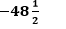Solutions:

(i) Given here,

A.P. = 10, 7, 4, …

Therefore, we can find,

First term, a = 10

Common difference, d = a2 − a= 7−10 = −3

As we know, for an A.P.,

an = a +(n−1)d

Putting the values;

a30 = 10+(30−1)(−3)

a30 = 10+(29)(−3)

a30 = 10−87 = −77

Hence, the correct answer is option C.

(ii) Given here,

A.P. = -3, -1/2, ,2 …

Therefore, we can find,

First term a = – 3

Common difference, d = a2 − a1 = (-1/2) -(-3)

⇒(-1/2) + 3 = 5/2

As we know, for an A.P.,

an = a+(n−1)d

Putting the values;

a11 = -3+(11-1)(5/2)

a11 = -3+(10)(5/2)

a11 = -3+25

a11 = 22

Hence, the answer is option B.

3. In the following APs find the missing term in the boxes.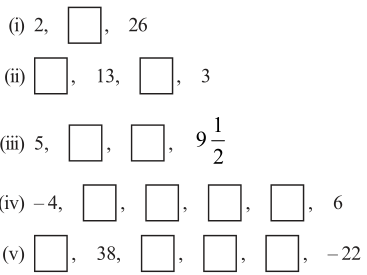Solutions:

(i) For the given A.P., 2,2 , 26

The first and third term are;

a = 2

a3 = 26

As we know, for an A.P.,

an = a+(n −1)d

Therefore, putting the values here,

a3 = 2+(3-1)d

26 = 2+2d

24 = 2d

d = 12

a2 = 2+(2-1)12

= 14

Therefore, 14 is the missing term.

(ii) For the given A.P., , 13, ,3

a2 = 13 and

a4 = 3

As we know, for an A.P.,

an = a+(n−1) d

Therefore, putting the values here,

a2 = a +(2-1)d

13 = a+d ………………. (i)

a4 = a+(4-1)d

3 = a+3d ………….. (ii)

On subtracting equation (i) from (ii), we get,

– 10 = 2d

d = – 5

From equation (i), putting the value of d,we get

13 = a+(-5)

a = 18

a3 = 18+(3-1)(-5)

= 18+2(-5) = 18-10 = 8

Therefore, the missing terms are 18 and 8 respectively.

(iii) For the given A.P.,

= 5 and

a4 = 19/2

As we know, for an A.P.,

an = a+(n−1)d

Therefore, putting the values here,

a4 = a+(4-1)d

19/2 = 5+3d

(19/2) – 5 = 3d

3d = 9/2

d = 3/2

a2 = a+(2-1)d

a2 = 5+3/2

a2 = 13/2

a3 = a+(3-1)d

a3 = 5+2×3/2

a3 = 8

Therefore, the missing terms are 13/2 and 8 respectively.

(iv) For the given A.P.,

a = −4 and

a6 = 6

As we know, for an A.P.,

an = a +(n−1) d

Therefore, putting the values here,

a6 = a+(6−1)d

6 = − 4+5d

10 = 5d

d = 2

a2 = a+d = − 4+2 = −2

a3 = a+2d = − 4+2(2) = 0

a4 = a+3d = − 4+ 3(2) = 2

a5 = a+4d = − 4+4(2) = 4

Therefore, the missing terms are −2, 0, 2, and 4 respectively.

(v) For the given A.P.,

a2 = 38

a6 = −22

As we know, for an A.P.,

an = a+(n −1)d

Therefore, putting the values here,

a2 = a+(2−1)d

38 = a+d ……………………. (i)

a6 = a+(6−1)d

−22 = a+5d …………………. (ii)

On subtracting equation (i) from (ii), we get

− 22 − 38 = 4d

−60 = 4d

d = −15

a = a2 − d = 38 − (−15) = 53

a3 = + 2= 53 + 2 (−15) = 23

a4 = a + 3d = 53 + 3 (−15) = 8

a5 = a + 4d = 53 + 4 (−15) = −7

Therefore, the missing terms are 53, 23, 8, and −7 respectively.

4. Which term of the A.P. 3, 8, 13, 18, … is 78?

Solutions:

Given the A.P. series as3, 8, 13, 18, …

First term, a = 3

Common difference, d = a2 − a1 = 8 − 3 = 5

Let the nth term of given A.P. be 78. Now as we know,

an = a+(n−1)d

Therefore,

78 = 3+(n −1)5

75 = (n−1)5

(n−1) = 15

n = 16

Hence, 16th term of this A.P. is 78.

5. Find the number of terms in each of the following A.P.

(i) 7, 13, 19, …, 205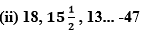Solutions:

(i) Given, 7, 13, 19, …, 205 is the A.P

Therefore

First term, a = 7

Common difference, d = a2 − a1 = 13 − 7 = 6

Let there are n terms in this A.P.

an = 205

As we know, for an A.P.,

an = a + (n − 1) d

Therefore, 205 = 7 + (− 1) 6

198 = (n − 1) 6

33 = (n − 1)

n = 34

Therefore, this given series has 34 terms in it.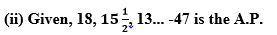First term, a = 18

Common difference, d = a2-a=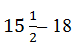d = (31-36)/2 = -5/2

Let there are n terms in this A.P.

an = -47

As we know, for an A.P.,

an = a+(n−1)d

-47 = 18+(n-1)(-5/2)

-47-18 = (n-1)(-5/2)

-65 = (n-1)(-5/2)

(n-1) = -130/-5

(n-1) = 26

n = 27

Therefore, this given A.P. has 27 terms in it.

6. Check whether -150 is a term of the A.P. 11, 8, 5, 2, …

Solution:

For the given series, A.P. 11, 8, 5, 2..

First term, a = 11

Common difference, d = a2a1 = 8−11 = −3

Let −150 be the nth term of this A.P.

As we know, for an A.P.,

an = a+(n−1)d

-150 = 11+(n -1)(-3)

-150 = 11-3n +3

-164 = -3n

n = 164/3

Clearly, n is not an integer but a fraction.

Therefore, – 150 is not a term of this A.P.

7. Find the 31st term of an A.P. whose 11th term is 38 and the 16th term is 73.

Solution:

Given that,

11th term, a11 = 38

and 16th term, a16 = 73

We know that,

an = a+(n−1)d

a11 = a+(11−1)d

38 = a+10d ………………………………. (i)

In the same way,

a16 = a +(16−1)d

73 = a+15d ………………………………………… (ii)

On subtracting equation (i) from (ii), we get

35 = 5d

d = 7

From equation (i), we can write,

38 = a+10×(7)

38 − 70 = a

a = −32

a31 = a +(31−1) d

= − 32 + 30 (7)

= − 32 + 210

= 178

Hence, 31st term is 178.

8. An A.P. consists of 50 terms of which 3rd term is 12 and the last term is 106. Find the 29th term.

Solution: Given that,

3rd term, a3 = 12

50th term, a50 = 106

We know that,

an = a+(n−1)d

a3 = a+(3−1)d

12 = a+2d ……………………………. (i)

In the same way,

a50 a+(50−1)d

106 = a+49d …………………………. (ii)

On subtracting equation (i) from (ii), we get

94 = 47d

d = 2 = common difference

From equation (i), we can write now,

12 = a+2(2)

a = 12−4 = 8

a29 = a+(29−1) d

a29 = 8+(28)2

a29 = 8+56 = 64

Therefore, 29th term is 64.

9. If the 3rd and the 9th terms of an A.P. are 4 and − 8 respectively. Which term of this A.P. is zero.
Solution:

Given that,

3rd term, a3 = 4

and 9th term, a9 = −8

We know that,

an = a+(n−1)d

Therefore,

a3 = a+(3−1)d

4 = a+2d ……………………………………… (i)

a9 = a+(9−1)d

−8 = a+8d ………………………………………………… (ii)

On subtracting equation (i) from (ii), we will get here,

−12 = 6d

d = −2

From equation (i), we can write,

4 = a+2(−2)

4 = a−4

a = 8

Let nth term of this A.P. be zero.

aa+(n−1)d

0 = 8+(n−1)(−2)

0 = 8−2n+2

2= 10

n = 5

Hence, 5th term of this A.P. is 0.

10. If 17th term of an A.P. exceeds its 10th term by 7. Find the common difference.

Solution:

We know that, for an A.P series;

an = a+(n−1)d

a17 = a+(17−1)d

a17 = a +16d

In the same way,

a10 = a+9d

As it is given in the question,

a17 − a10 = 7

Therefore,

(a +16d)−(a+9d) = 7

7d = 7

d = 1

Therefore, the common difference is 1.

11. Which term of the A.P. 3, 15, 27, 39,.. will be 132 more than its 54th term?

Solution:

Given A.P. is 3, 15, 27, 39, …

first term, a = 3

common difference, d = a2 − a1 = 15 − 3 = 12

We know that,

an = a+(n−1)d

Therefore,

a54 = a+(54−1)d

⇒3+(53)(12)

⇒3+636 = 639

a54 = 639+132=771

We have to find the term of this A.P. which is 132 more than a54, i.e.771.

Let nth term be 771.

an = a+(n−1)d

771 = 3+(n −1)12

768 = (n−1)12

(n −1) = 64

n = 65

Therefore, 65th term was 132 more than 54th term.

Or another method is;

Let nth term be 132 more than 54th term.

n = 54 + 132/2

= 54 + 11 = 65th term

12. Two APs have the same common difference. The difference between their 100th term is 100, what is the difference between their 1000th terms?

Solution:

Let, the first term of two APs be a1 and a2 respectively

And the common difference of these APs be d.

For the first A.P.,we know,

an = a+(n−1)d

Therefore,

a100 = a1+(100−1)d

a1 + 99d

a1000 = a1+(1000−1)d

a1000 = a1+999d

For second A.P., we know,

an = a+(n−1)d

Therefore,

a100 = a2+(100−1)d

a2+99d

a1000 = a2+(1000−1)d

a2+999d

Given that, difference between 100th term of the two APs = 100

Therefore, (a1+99d) − (a2+99d) = 100

a1a2 = 100……………………………………………………………….. (i)

Difference between 1000th terms of the two APs

(a1+999d) − (a2+999d) = a1a2

From equation (i),

This difference, a1a= 100

Hence, the difference between 1000th terms of the two A.P. will be 100.

13. How many three digit numbers are divisible by 7?

Solution:

First three-digit number that is divisible by 7 are;

First number = 105

Second number = 105+7 = 112

Third number = 112+7 =119

Therefore, 105, 112, 119, …

All are three digit numbers are divisible by 7 and thus, all these are terms of an A.P. having first term as 105 and common difference as 7.

As we know, the largest possible three-digit number is 999.

When we divide 999 by 7, the remainder will be 5.

Therefore, 999-5 = 994 is the maximum possible three-digit number that is divisible by 7.

Now the series is as follows.

105, 112, 119, …, 994

Let 994 be the nth term of this A.P.

first term, a = 105

common difference, d = 7

an = 994

n = ?

As we know,

an = a+(n−1)d

994 = 105+(n−1)7

889 = (n−1)7

(n−1) = 127

n = 128

Therefore, 128 three-digit numbers are divisible by 7.

14. How many multiples of 4 lie between 10 and 250?

Solution:

The first multiple of 4 that is greater than 10 is 12.

Next multiple will be 16.

Therefore, the series formed as;

12, 16, 20, 24, …

All these are divisible by 4 and thus, all these are terms of an A.P. with first term as 12 and common difference as 4.

When we divide 250 by 4, the remainder will be 2. Therefore, 250 − 2 = 248 is divisible by 4.

The series is as follows, now;

12, 16, 20, 24, …, 248

Let 248 be the nth term of this A.P.

first term, a = 12

common difference, d = 4

an = 248

As we know,

an = a+(n−1)d

248 = 12+(n-1)×4

236/4 = n-1

59  = n-1

n = 60

Therefore, there are 60 multiples of 4 between 10 and 250.

15. For what value of n, are the nth terms of two APs 63, 65, 67, and 3, 10, 17, … equal?

Solution:

Given two APs as; 63, 65, 67,… and 3, 10, 17,….

Taking first AP,

63, 65, 67, …

First term, a = 63

Common difference, d = a2−a1 = 65−63 = 2

We know, nth term of this A.P. = an = a+(n−1)d

an= 63+(n−1)2 = 63+2n−2

an = 61+2n ………………………………………. (i)

Taking second AP,

3, 10, 17, …

First term, a = 3

Common difference, d = a2 − a1 = 10 − 3 = 7

We know that,

nth term of this A.P. = 3+(n−1)7

an = 3+7n−7

an = 7n−4 ……………………………………………………….. (ii)

Given, nth term of these A.P.s are equal to each other.

Equating both these equations, we get,

61+2n = 7n−4

61+4 = 5n

5n = 65

n = 13

Therefore, 13th terms of both these A.P.s are equal to each other.

16. Determine the A.P. whose third term is 16 and the 7th term exceeds the 5th term by 12.

Solutions:

Given,

Third term, a3 = 16

As we know,

a +(3−1)d = 16

a+2d = 16 ………………………………………. (i)

It is given that, 7th term exceeds the 5th term by 12.

a7 − a5 = 12

[a+(7−1)d]−[+(5−1)d]= 12

(a+6d)−(a+4d) = 12

2d = 12

d = 6

From equation (i), we get,

a+2(6) = 16

a+12 = 16

a = 4

Therefore, A.P. will be4, 10, 16, 22, …

17. Find the 20th term from the last term of the A.P. 3, 8, 13, …, 253.

Solution:

Given A.P. is3, 8, 13, …, 253

Common difference, d= 5.

Therefore, we can write the given AP in reverse order as;

253, 248, 243, …, 13, 8, 5

Now for the new AP,

first term, a = 253

and common difference, d = 248 − 253 = −5

n = 20

Therefore, using nth term formula, we get,

a20 = a+(20−1)d

a20 = 253+(19)(−5)

a20 = 253−95

a = 158

Therefore, 20th term from the last term of the AP 3, 8, 13, …, 253.is 158.

18. The sum of 4th and 8th terms of an A.P. is 24 and the sum of the 6th and 10th terms is 44. Find the first three terms of the A.P.

Solution:

We know that, the nth term of the AP is;

an = a+(n−1)d

a4 = a+(4−1)d

a4 = a+3d

In the same way, we can write,

a8 = a+7d

a6 = a+5d

a10 = a+9d

Given that,

a4+a8 = 24

a+3d+a+7d = 24

2a+10d = 24

a+5d = 12 …………………………………………………… (i)

a6+a10 = 44

a +5d+a+9d = 44

2a+14d = 44

a+7d = 22 …………………………………….. (ii)

On subtracting equation (i) from (ii), we get,

2d = 22 − 12

2d = 10

d = 5

From equation (i), we get,

a+5d = 12

a+5(5) = 12

a+25 = 12

a = −13

a2 = a+d = − 13+5 = −8

a3 = a2+d = − 8+5 = −3

Therefore, the first three terms of this A.P. are −13, −8, and −3.

19. Subba Rao started work in 1995 at an annual salary of Rs 5000 and received an increment of Rs 200 each year. In which year did his income reach Rs 7000?

Solution:

It can be seen from the given question, that the incomes of Subba Rao increases every year by Rs.200 and hence, forms an AP.

Therefore, after 1995, the salaries of each year are;

5000, 5200, 5400, …

Here, first term, a = 5000

and common difference, d = 200

Let after nth year, his salary be Rs 7000.

Therefore, by the nth term formula of AP,

an = a+(n−1) d

7000 = 5000+(n−1)200

200(n−1)= 2000

(n−1) = 10

n = 11

Therefore, in 11th year, his salary will be Rs 7000.

20. Ramkali saved Rs 5 in the first week of a year and then increased her weekly saving by Rs 1.75. If in the nth week, her weekly savings become Rs 20.75, find n.

Solution:

Given that, Ramkali saved Rs.5 in first week and then started saving each week by Rs.1.75.

Hence,

First term, a = 5

and common difference, d = 1.75

Also given,

a= 20.75

Find, n = ?

As we know, by the nth term formula,

an = a+(n−1)d

Therefore,

20.75 = 5+(n -1)×1.75

15.75 = (n -1)×1.75

(n -1) = 15.75/1.75 = 1575/175

= 63/7 = 9

n -1 = 9

n = 10

Hence, n is 10.

### Access other exercise solutions of class 10 Maths Chapter 5- Arithmetic progression

Exercise 5.1– 4 questions 1 MCQ and 3 descriptive type questions
Exercise 5.3– 20 Questions 3 fill in the blanks, 4 daily life examples, and 13 descriptive type questions
Exercise 5.4 5 Questions- 5 Long answer questions